Ch 8. Strain Analysis Multimedia Engineering Mechanics PlaneStrain Mohr's Circlefor Strain StrainGages
 Chapter 1. Stress/Strain 2. Torsion 3. Beam Shr/Moment 4. Beam Stresses 5. Beam Deflections 6. Beam-Advanced 7. Stress Analysis 8. Strain Analysis 9. Columns Appendix Basic Math Units Basic Equations Sections Material Properties Structural Shapes Beam Equations Search eBooks Dynamics Fluids Math Mechanics Statics Thermodynamics Author(s): Kurt Gramoll ©Kurt GramollMECHANICS - CASE STUDY SOLUTION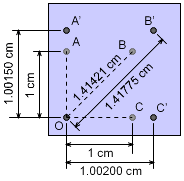Distance Between Points Before

The distance between points A, B, C and O are measured using a laser device before and after the bracket is loaded. The results are listed in the table below.

 Distance (cm) Pt 1 Pt 2 Initial Final O A 1.0 1.00150 O B 1.41421 1.41775 O C 1.0 1.00200

These displacements can be used to find the normal strain in the direction of OA, OB, and OC. Then, the strains can be analyzed using the strain transformation equations. Finally, the maximum shear strain can be determined.

Normal Strains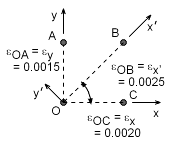Normal Strain Between Points

Since the distance before and after loading are known, the normal strains can be determined as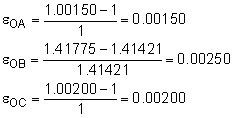The εOA and εOC are in the direction of the y-axis and x-axis, respectively. Also, the strain εOB is in the direction of the new rotated coordinate x´. This gives

εOA = εy = 0.00150 = 1,500 μ

εOB = ε = 0.00250 = 2,500 μ

εOC = εx = 0.00200 = 2,000 μ

Since strain is generally a very small number, the symbol μ "micro-strain" is commonly used to represent 10-6. This symbol does not represent units, and strain is still unit-less.

Rotating Strains

The stress element needs to be rotated 45o in the positive direction. Using the stress transformation equations, the stresses in the new x'-y' coordinate system will be,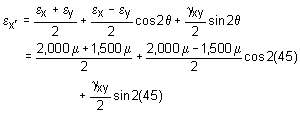2,500 μ = 1,750 μ + 0 + γxy/2

γxy = 750 μ = 0.000750

Maximum Shear Strain

The maximum shear strain can be found by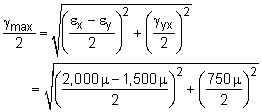γmax = 450.7μ = 0.0004507

The maximum shear strain will on a plane that is rotated θγ-max. That angle is,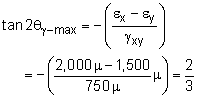θγ-max = -16.85o

Practice Homework and Test problems now available in the 'Eng Mechanics' mobile app
Includes over 400 problems with complete detailed solutions.
Available now at the Google Play Store and Apple App Store.